# Lines and Angles Chapter Exam

Exam Instructions:

Choose your answers to the questions and click 'Next' to see the next set of questions. You can skip questions if you would like and come back to them later with the yellow "Go To First Skipped Question" button. When you have completed the practice exam, a green submit button will appear. Click it to see your results. Good luck!

### Page 1

#### Question 1 1. What is the relationship between Angle 1 and Angle 7?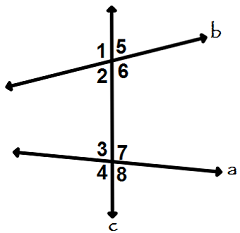#### Question 2 2. In triangle GBN, Angle G is seven more than three times a number, Angle B is five more than four times the number, and Angle N is twelve less than five times the number. Solve for the measure of Angle G.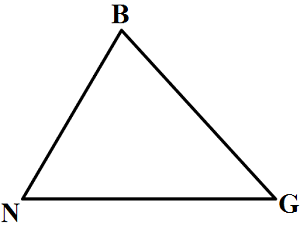#### Question 4 4. What is the relationship between Angle 2 and Angle 7?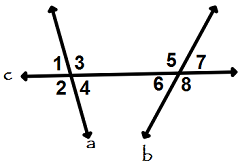#### Question 5 5. Given the figure below, Angle 3 = 140y and Angle 5 = 80y + 70. Determine the value of y.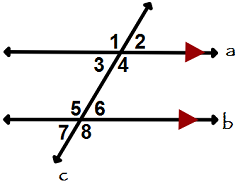### Page 2

#### Question 6 6. Which two lines are parallel?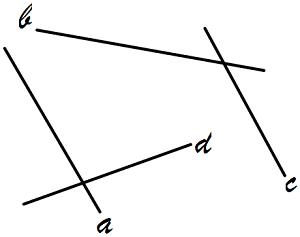#### Question 8 8. Given the triangle PQE, calculate the measure of Angle Q.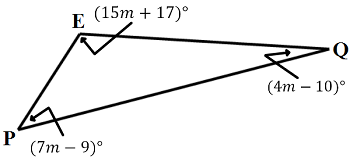#### Question 9 9. In the figure below, Angle 13 = 10y and Angle 11 = 3y + 11. Determine the measure of Angle 16.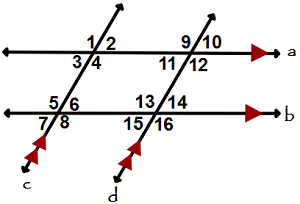### Page 3

#### Question 11 11. Given the triangle ABC, solve for the value of y.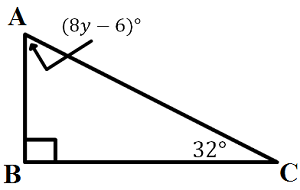#### Question 12 12. Consider the figure below, where Angle 4 = 12m - 4 Angle 5 = 10m + 20 Calculate the measure of Angle 5.#### Question 13 13. What is the relationship between Angle 4 and Angle 1?#### Question 14 14. Which line is the transversal?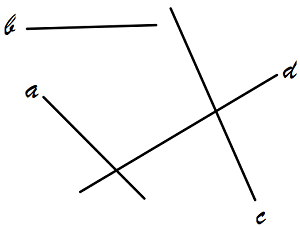#### Question 15 15. In the figure below, Angle 7 = 15x and Angle 10 = 9x + 30. Determine the measure of Angle 10.#### Lines and Angles Chapter Exam Instructions

Choose your answers to the questions and click 'Next' to see the next set of questions. You can skip questions if you would like and come back to them later with the yellow "Go To First Skipped Question" button. When you have completed the practice exam, a green submit button will appear. Click it to see your results. Good luck!

Support